##### Actions

(diff) ← Older revision | Latest revision (diff) | Newer revision → (diff)

Szegö quadrature formulas are the analogues on the unit circle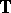in the complex plane of the Gauss quadrature formulas on an interval (cf. also Gauss quadrature formula). They approximate the integral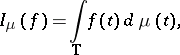whereandis a positive measure on, by a quadrature formula of the formOne cannot take the zeros of the Szegö polynomialsas nodes (as in Gaussian formulas), because these are all in the open unit disc(cf. also Szegö polynomial). Therefore, the para-orthogonal polynomials are introduced as, where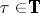and. These are orthogonal to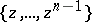and havesimple zeros, which are on. The Szegö quadrature formula then takes as nodes the zeros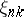,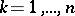, of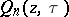, and as weights the Christoffel numbers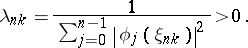The result is a quadrature formula with a maximal domain of validity in the set of Laurent polynomials, i.e., the formula is exact for all trigonometric polynomials in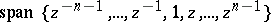, a space of dimension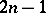, which is the maximal dimension possible with a quadrature formula of this form.

The Szegö quadrature formulas were introduced in [a2]. The underlying ideas have been generalized from polynomials to rational functions. See [a1].

How to Cite This Entry: